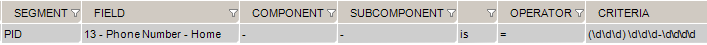# Validate Field is a valid phone number

This tutorial explains how to build a Segment/Field rule validating that a field contains a valid phone number.

In this example, we’ll validate that PID.13 (Phone Number – Home) contains a phone number.

1. In the inbound HL7 task, select the Validation tab
2. Select the Segment/Field Validation tab
3. Add the rule: PID.13 is matching regex (\d\d\d) \d\d\d-\d\d\d\dThis rule means that:

• Area code (\d\d\d): 3 digits between parentheses
• Phone number (\d\d\d-\d\d\d\d):  3 digits followed by a dash and 4 digits